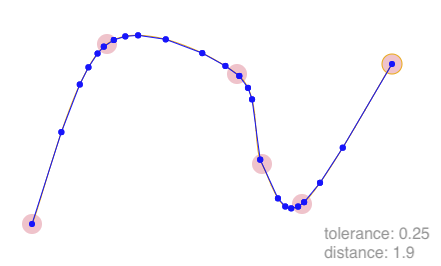# points-on-curve

Estimate points on a bezier curve or a set of connexted bezier curves

## Stats

StarsIssuesVersionUpdatedCreatedSize
points-on-curve
1.0.12 months ago4 years agopoints-on-curve
This package calculate the points on a curve with a certain tolerance. It can also simplify the shape to use fewer points. This can really be useful when estimating lines/polygons for curves in WebGL or for Hit/Collision detections.

## Install

From npm
``npm install --save points-on-curve``

The package is distributed as an ES6 module.

## API

### pointsOnBezierCurves(points: Point, tolerance?: number, distance?: number): Point

You pass in the points representing a bezier curve. Each point is an array of two numbers e.g. `[100, 123]`.
The points can also be a set of continuous curves where the last poing on the `Nth` curve acts as the first point of the next.
``````import { pointsOnBezierCurves } from 'points-on-curve';

const curve = [[70,240],[145,60],[275,90],[300,230]];
const points = pointsOnBezierCurves(curve);
// plotPoints(points);``````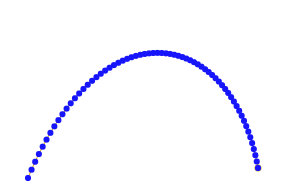Same can be rendered with more tolerance (default value is 0.15):
``const points = pointsOnBezierCurves(curve, 0.7);``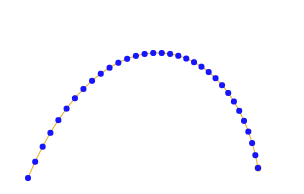Note that this method does not accept the number of points to render, but takes in a tolerance level which allows for better distribution of points.
The value of tolerance can be between 0 and 1. It is used to decide how many points are needed in a section of the curve. The algorithm determined the flatness of a section of the curve and compares it to the tolerance level, if less flat, the segment gets further divided into 2 segments.

#### Simplifying path

Based on the tolerance alone, this algorithm nicely provides enough points to represent a curve. It does not, however, efficiently get rid of unneeded points. The second optional argument in function, distance helps with that. If a `distance` value is provided, the method uses the Ramer–Douglas–Peucker algorithm to reduce the points.
``const points = pointsOnBezierCurves(curve, 0.2, 0.15);``

Following are the points generated with distance values of `0.15`, `0.75`, `1.5`, and `3.0`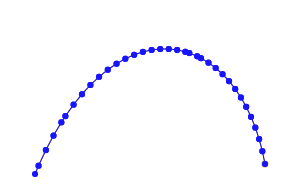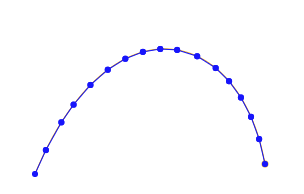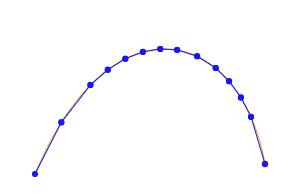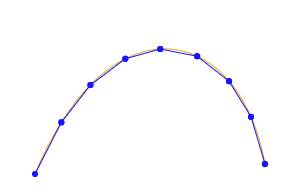### curveToBezier(pointsIn: Point): Point

Sometimes it's hard to think of shape as a set of cubic bezier curves, each curve with 2 controls points. It is simple to just think of them as a curve passing through a set of points.
This method turns those set of points to a set of points representing bezier curves.
``````import { curveToBezier } from 'points-on-curve/lib/curve-to-bezier.js';

const curvePoints = [
[20, 240],
[95, 69],
[225, 90],
[250, 180],
[290, 220],
[380, 80],
];
const bcurve = curveToBezier(curvePoints);
// .. Plot bcurve``````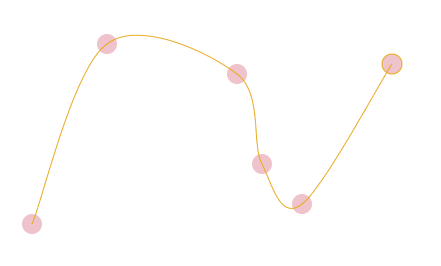Now that we have bezier points, these could be passed to `pointsOnBezierCurves` function to get the points on the curve